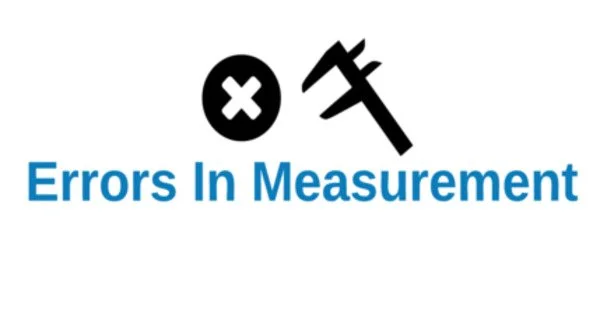Physics

# Errors in MeasurementThe foundation of any experimental study is measurement. Without constantly raising criteria for measuring precision, many of the greatest scientific discoveries would not have been feasible. To as accurately as possible determine a physical quantity is the goal of every experiment. But every measurement contains some inaccuracy, which could be brought on by the observer, the device, or even both. Measurement error is the distinction between a quantity’s actual value and its estimated value. An mistake could be either positive or negative. Error is defined as the difference between the measured quantity and the true value.

The uncertainty in the measurement of a physical quantity is called error. It is the difference between the true value and the measured value of the physical quantity. Errors may be classified into many categories.

(i) Constant errors

It is the same error repeated every time in a series of observations. Constant error is due to faulty calibration of the scale in the measuring instrument. In order to minimize constant error, measurements are made by different possible methods and the mean value so obtained is regarded as the true value.

(ii) Systematic errors

These are errors which occur due to a certain pattern or system. These errors can be minimized by identifying the source of error. Instrumental errors, personal errors due to individual traits and errors due to external sources are some of the systematic errors.

(iii) Gross errors

Gross errors arise due to one or more than one of the following reasons.

(1) Improper setting of the instrument.

(2) Wrong recordings of the observation.

(3) Not taking into account sources of error and precautions.

(4) Usage of wrong values in the calculation.

Gross errors can be minimized only if the observer is very careful in his observations and sincere in his approach.

(iv) Random errors

It is very common that repeated measurements of a quantity give values which are slightly different from each other. These errors have no set pattern and occur in a random manner. Hence they are called random errors. They can be minimized by repeating the measurements many times and taking the arithmetic mean of all the values as the correct reading.

The most common way of expressing an error is percentage error.

If the accuracy in measuring a quantity x is Δx, then the percentage error in x is given by (Δx/x) × 100 %.